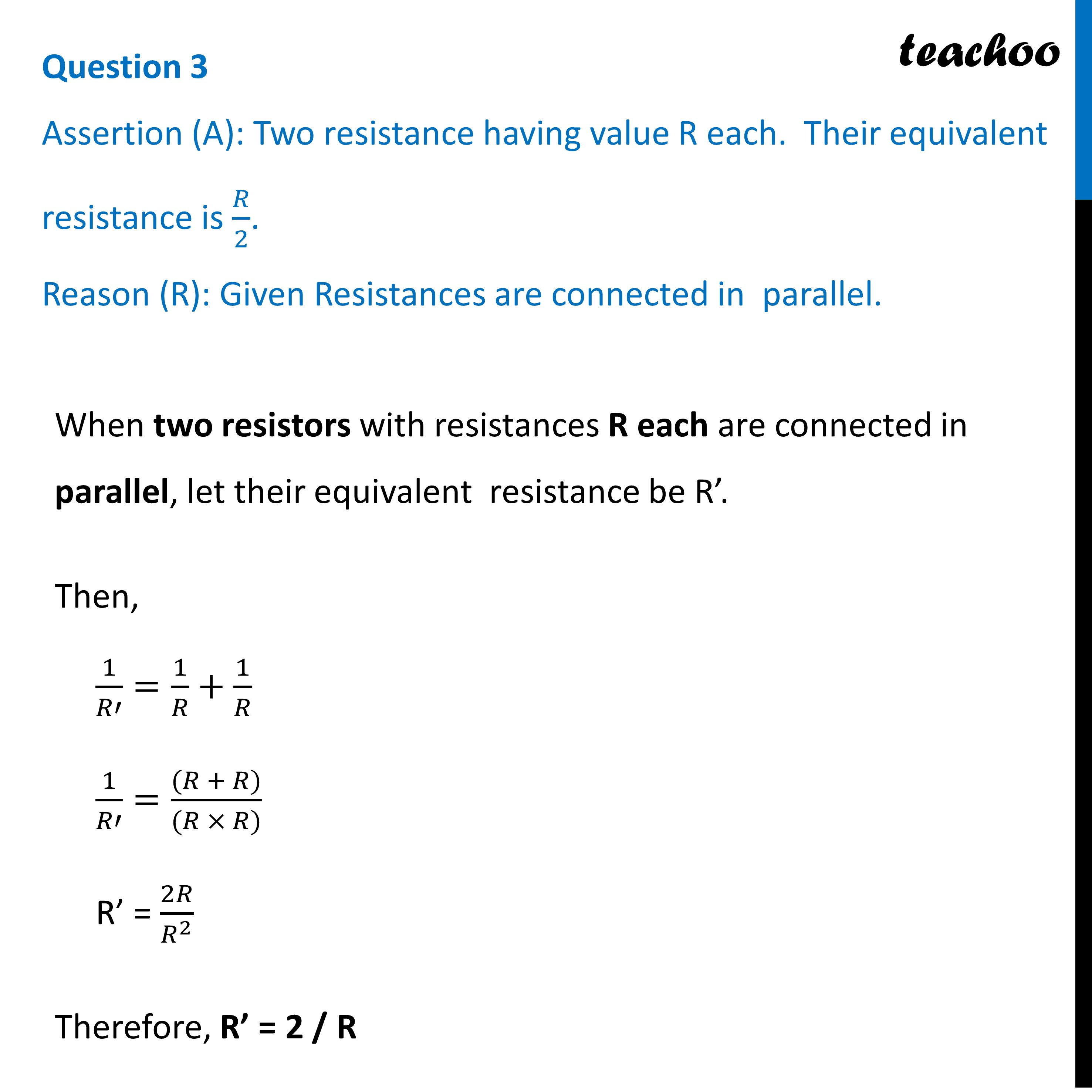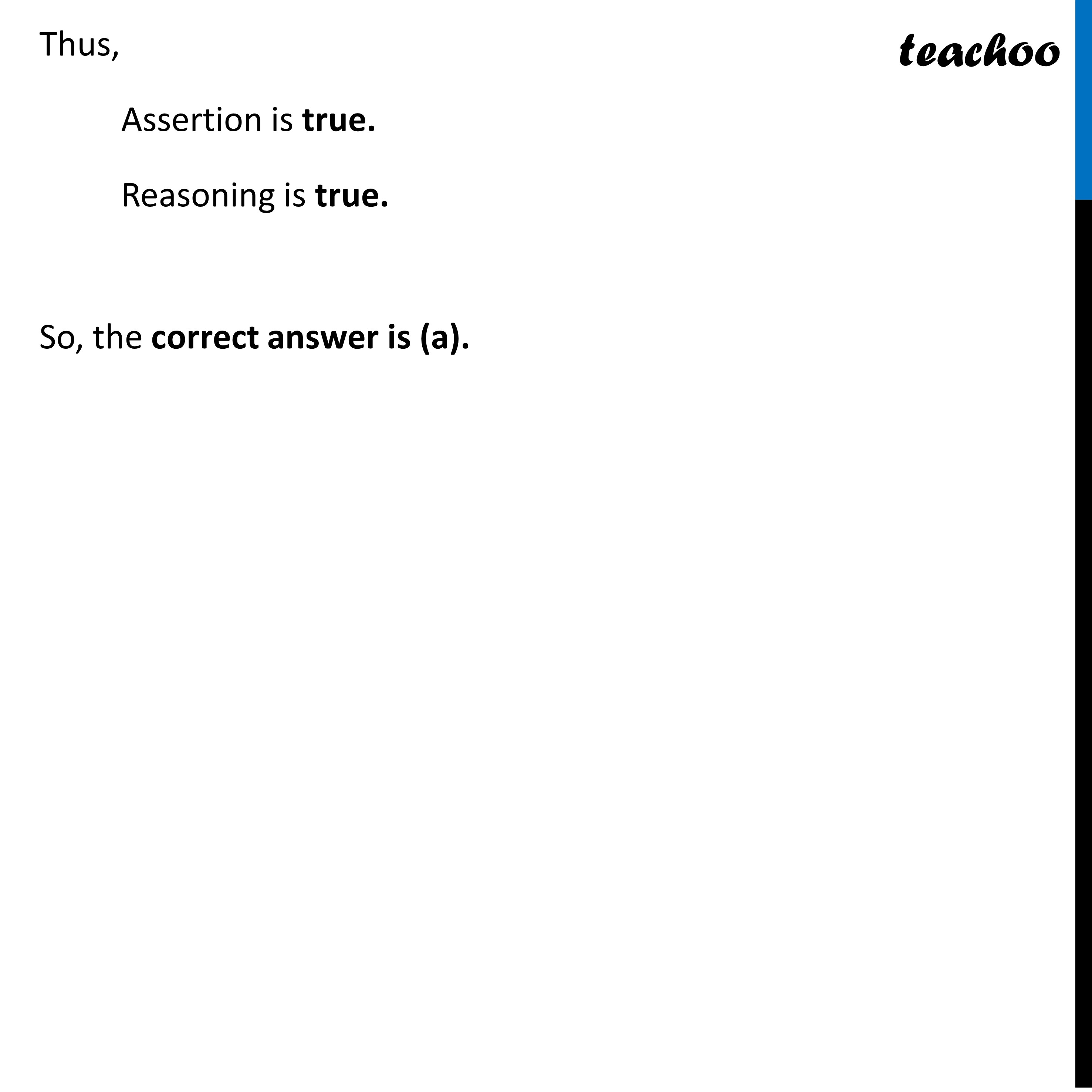Assertion Reasoning questions (MCQ)

Class 10
Chapter 12 Class 10 - Electricity

## Reason (R): Given Resistances are connected in parallel.Learn in your speed, with individual attention - Teachoo Maths 1-on-1 Class

### Transcript

Question 3 Assertion (A): Two resistance having value R each. Their equivalent resistance is 𝑅/2. Reason (R): Given Resistances are connected in parallel. When two resistors with resistances R each are connected in parallel, let their equivalent resistance be R’. Then, 1/𝑅′=1/𝑅+1/𝑅 1/𝑅′=((𝑅 + 𝑅))/((𝑅 × 𝑅)) R’ = 2𝑅/𝑅^2 Therefore, R’ = 2 / R Thus, Assertion is true. Reasoning is true. So, the correct answer is (a).